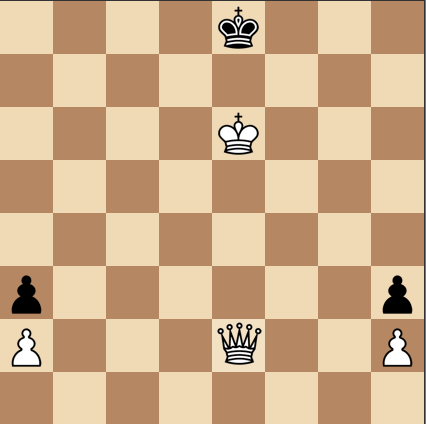# Fastest checkmate

Logic Level 3

In the position below, how can White give the fastest checkmate? (Assume both players are moving optimally.)

The target square of the first move can be represented as a coordinate $(x,y)$, where the bottom left corner is at $(0,0)$. Input the product of $x$ and $y$ as your answer.×